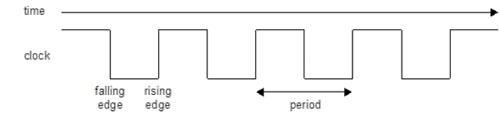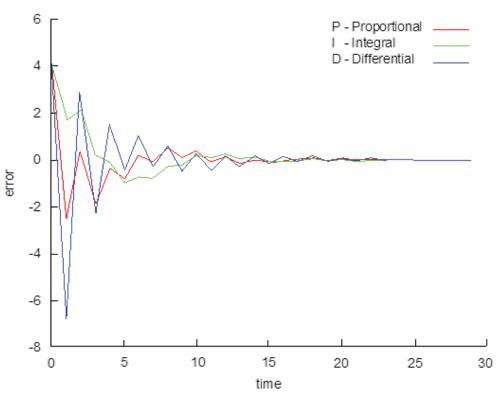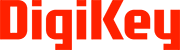# Handling Clocks in Software

Digi-Key Electronics の提供

Clocks are standard concepts for hardware designers but less familiar to software engineers. However, in embedded programming (and particularly real-time embedded programming) software developers have to handle clocks in their software programs. This article discusses the basics of software-based clocks.

What makes a clock

A clock is a signal that alternates with a fixed frequency.Figure 1: A clock signal.

The period of the clock is the time between consecutive rising edges of the signal. The frequency is the rate at which rising edges occur (i.e., 1/period). The period or frequency characterizes the clock. In software, to keep track of a clock you need to store just one of these values. In this article, the examples characterize the clock with its period since this makes them easier to code. For the sake of exposition, let’s use a floating point type for representing it (e.g., the C double type). In practice, any type that can represent time can be used. A fixed point representation is often used.

Using a clock

Once a clock frequency is available in software you can easily multiply or divide the clock simply by multiplying or dividing the period. The information about the clock can be delivered to a remote system to recreate remotely, or to control a stream of data that is to be transported in sync with the clock.

Outputting a clock

To output the clock you need to bit-bash an output port alternately high and low. The following C-style pseudo-code shows the idea:

double t;
int val = 0;
t = get_current_time();
while (1) {
t += period/2;
output_at_time(t, val);
val = ~val;
}

Here get_current_time gets the current time and output_at_time outputs a value to a port at the specified time. However, these functions are accurate only to some resolution inherent in the software system. The frequency of the output clock is quantized to that resolution, meaning the frequency can be quite far off the required value. You can improve this by keeping track of the error caused by this quantization and adjusting as you go along. The following code shows this:

double t;
int val = 0;
double hi = floor((period/2)/resolution)*resolution;
double lo = (period/2) -hi;
double err = 0;

t = get_time();
while (1) {
t += hi;
err += lo;
if (err >= resolution) {
err -= resolution;
t += 1;
}
output_at_time(t, val);
val = ~val;
}

This algorithm keeps track of the error and when the error gets big enough to incorporate into the output, it does so. With this method, the output clock will have the correct frequency but the quantization will still cause an observable jitter on the clock.

Recovering a clock

Sometimes you need to recover the clock from an incoming signal so you can use it as a base for other processing in the system.

Matching the frequency

The simplest thing to do is match the frequency of the incoming clock. Over a particular period, count the number of rising edges of the clock and then calculate the period per rising edge:

period = sample_period / ticks_in;

The incoming clock varies its frequency over time so you have to resample regularly.

Using a feedback loop

Sometimes just matching the frequency of a clock is not enough. The small mismatches and adjustments add up over time causing your internal notion of a clock and the actual clock to drift over a long period. The number of ticks from the recovered clock can be different than the number of ticks of the original clock. To address this, you need to continually adjust for the accumulated error between the clocks, a task that can be done using a PID control loop.¹

Suppose you want to match an outgoing clock to an incoming clock. The idea is to adjust the outgoing clock period at regular intervals. At each adjustment point, look at the number of ticks since the last adjustment of the incoming clock (ticks_in) and the number of ticks since the last adjustment of the outgoing clock (ticks_out). The difference between these is the proportional error of the clock.

From the proportional error, you can also calculate the integral (or accumulated) error and the differential error. The period is then adjusted based on these values to move the clock period towards the correct value. Over time the algorithm homes in on a fixed point and the proportional error tends towards zero. The following code can be used to adjust the period at each update:

P = ticks_out -ticks_in;
I = I + P;
D = P -prevP;
period = period + Kp * P + Ki * I + Kd * D;

The setting of the constants Kp, Ki and Kd affects how quickly the algorithm will settle and how much perturbation in the input clock it can handle. There is a trove of methods on calculating these constants correctly for your application that are not discussed here but a good starting point is the Wikipedia page on PID control loops.

The following graph shows a typical progression of the error of such an algorithm over time.Figure 2: PID Error Progression.
Why?

To a hardware engineer, the outcome of the previous section is hardly outstanding. A clock has been routed in a rather complex manner. So why do you need to bring clocks into the software domain? One reason is that with the clock as a logical entity in software, you can analyze it and manipulate it. For example, you can fractionally multiply the clock to be used elsewhere or you can report its frequency to some higher-level application.

However, one big application is that you can transmit a clock to another part of the system and recover it via a digital-only transport without the need to explicitly transport the clock. For example, the clock can be transported over a USB bus or an Ethernet network. This brings a wealth of benefits in terms of connectivity and flexibility that would be severely limited if you had to explicitly connect every clock signal in the system.

To recover a clock remotely, you need to transmit the feedback information (e.g., the tick counts). This counting over a period of time still requires a common time base, so all parts of the system must have the same sense of global time. How this is done is outside the scope of this article, but for bus-based systems (such as USB or Firewire) the bus may carry a global clock. For more loosely coupled systems such as Ethernet or other packet switched networks, a global clock recovery protocol is required such as IEEE 1588.²

References### 著者について

Dave Lacey

Article authored by Dave Lacey, Technical Director of Software Tools, XMOS.

### 出版者について

Digi-Key Electronics

ミネソタ州シーフリバーフォールズに拠点を置くDigi-Key Electronicsは、試作および設計段階、量産段階のいずれにおいても、電子部品を卓越したサービスとともにグローバルに提供し、Digi-Keyでは、750社以上の一流メーカーから提供される600万点以上の製品を取り扱っている。